Italian Charms Stamped Necklaces Welcome to our Italian Charm Jewelry ShopEnamel Italian CharmsAnimals: Land
Animals: Water
Animals: Cats
Animals: Dogs
Animals: Birds
Animals: Horses
Around the House
Baby
Bugs
California Charms Pg 1
California Charms Pg 2
California Charms Pg 3
Cartoon Charms
Casino
Celebrations
Charm Chicks
Colored Hearts
Cowboy
Dates
Easter
Smiley Faces
Family: General
Family: Mom
Fantasy
Flags: Countries
Flags: States
Flip Flops
Flowers
Food & Drinks
Fruits & Veggies
Gold Charms
Halloween

Hats
Holiday/Christmas
In Memory
Irish/St Patricks
Jobs
Love & Hearts
Make Up/Accessories
Military
Miscellaneous
Moon and Stars
Motorcycles
Music/Instruments
Nicknames
Ocean
Patriotic
Places & Landmarks
Planes/Trains/Automobiles
Red Hearts
Religious/Angels
Ribbons
Scenes
School
Sports: Individual
Sports: Team
Sports: Pro Teams
State Hearts
Words General
Words I Love
Clearance

Italian Charm Watches
All standard size watches come with an Italian Charm bracelet attached. The wider "MEGA" watches connect to two bracelets, which are not included with those particular watch styles. For a limited time ALL watches are under \$9 and as low as \$4.88 each in bulk!
Round Watches
Rectangular Watch
 1 at 8.88 2 at 7.88 3 at 6.88 6 at 5.88 12 at 4.88
 1 at 8.88 2 at 7.88 3 at 6.88 6 at 5.88 12 at 4.88
 1 at 8.88 2 at 7.88 3 at 6.88 6 at 5.88 12 at 4.88
 1 at 8.88 2 at 7.88 3 at 6.88 6 at 5.88 12 at 4.88
CZ Heart Watches
 1 at 8.88 2 at 7.88 3 at 6.88 6 at 5.88 12 at 4.88
 1 at 8.88 2 at 7.88 3 at 6.88 6 at 5.88 12 at 4.88
 1 at 8.88 2 at 7.88 3 at 6.88 6 at 5.88 12 at 4.88
 1 at 8.88 2 at 7.88 3 at 6.88 6 at 5.88 12 at 4.88
Long Rectangle Watches
 1 at 8.88 2 at 7.88 3 at 6.88 6 at 5.88 12 at 4.88
 1 at 8.88 2 at 7.88 3 at 6.88 6 at 5.88 12 at 4.88
 1 at 8.88 2 at 7.88 3 at 6.88 6 at 5.88 12 at 4.88
 1 at 8.88 2 at 7.88 3 at 6.88 6 at 5.88 12 at 4.88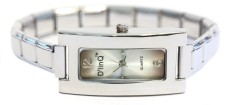WW111gray Gray Long Italian Charm Watch Silver Color Band
 1 at 8.88 2 at 7.88 3 at 6.88 6 at 5.88 12 at 4.88
 1 at 8.88 2 at 7.88 3 at 6.88 6 at 5.88 12 at 4.88
 1 at 8.88 2 at 7.88 3 at 6.88 6 at 5.88 12 at 4.88
 1 at 8.88 2 at 7.88 3 at 6.88 6 at 5.88 12 at 4.88
Gold Trim Square Watches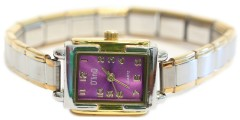WG104fuchsia Fuchsia Rectangle Italian Charm Watch Gold Color TrimWG104lavender Lavender Rectangle Italian Charm Watch Gold Color Trim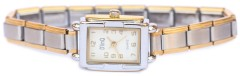WG104white White Rectangle Italian Charm Watch Gold Color Trim

 1 at 8.88 2 at 7.88 3 at 6.88 6 at 5.88 12 at 4.88
 1 at 8.88 2 at 7.88 3 at 6.88 6 at 5.88 12 at 4.88
 1 at 8.88 2 at 7.88 3 at 6.88 6 at 5.88 12 at 4.88
 1 at 8.88 2 at 7.88 3 at 6.88 6 at 5.88 12 at 4.88
Small Round Watches
Gold Trim Oval Watch
Heart Watch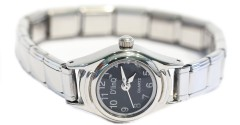WW106black Black Small Round Italian Charm Watch Silver Color Band
 1 at 8.88 2 at 7.88 3 at 6.88 6 at 5.88 12 at 4.88
 1 at 8.88 2 at 7.88 3 at 6.88 6 at 5.88 12 at 4.88
 1 at 8.88 2 at 7.88 3 at 6.88 6 at 5.88 12 at 4.88
 1 at 8.88 2 at 7.88 3 at 6.88 6 at 5.88 12 at 4.88
More Heart Italian Charm Watches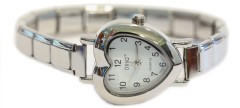WW211white White Heart Italian Charm Watch
 1 at 8.88 2 at 7.88 3 at 6.88 6 at 5.88 12 at 4.88
 1 at 8.88 2 at 7.88 3 at 6.88 6 at 5.88 12 at 4.88
 1 at 8.88 2 at 7.88 3 at 6.88 6 at 5.88 12 at 4.88
 1 at 8.88 2 at 7.88 3 at 6.88 6 at 5.88 12 at 4.88
Square Bling Watches
MEGA Watches Connects 2 Charm Bracelets
 1 at 8.88 2 at 7.88 3 at 6.88 6 at 5.88 12 at 4.88
 1 at 8.88 2 at 7.88 3 at 6.88 6 at 5.88 12 at 4.88
 1 at 8.88 2 at 7.88 3 at 6.88 6 at 5.88 12 at 4.88
 1 at 8.88 2 at 7.88 3 at 6.88 6 at 5.88 12 at 4.88
13mm Watches These are 1.5 Times Wider than Standard 9mm Watches

\$15 sale \$4.88 in any quantity

\$15 sale \$4.88 in any quantity

\$15 sale \$4.88 in any quantity